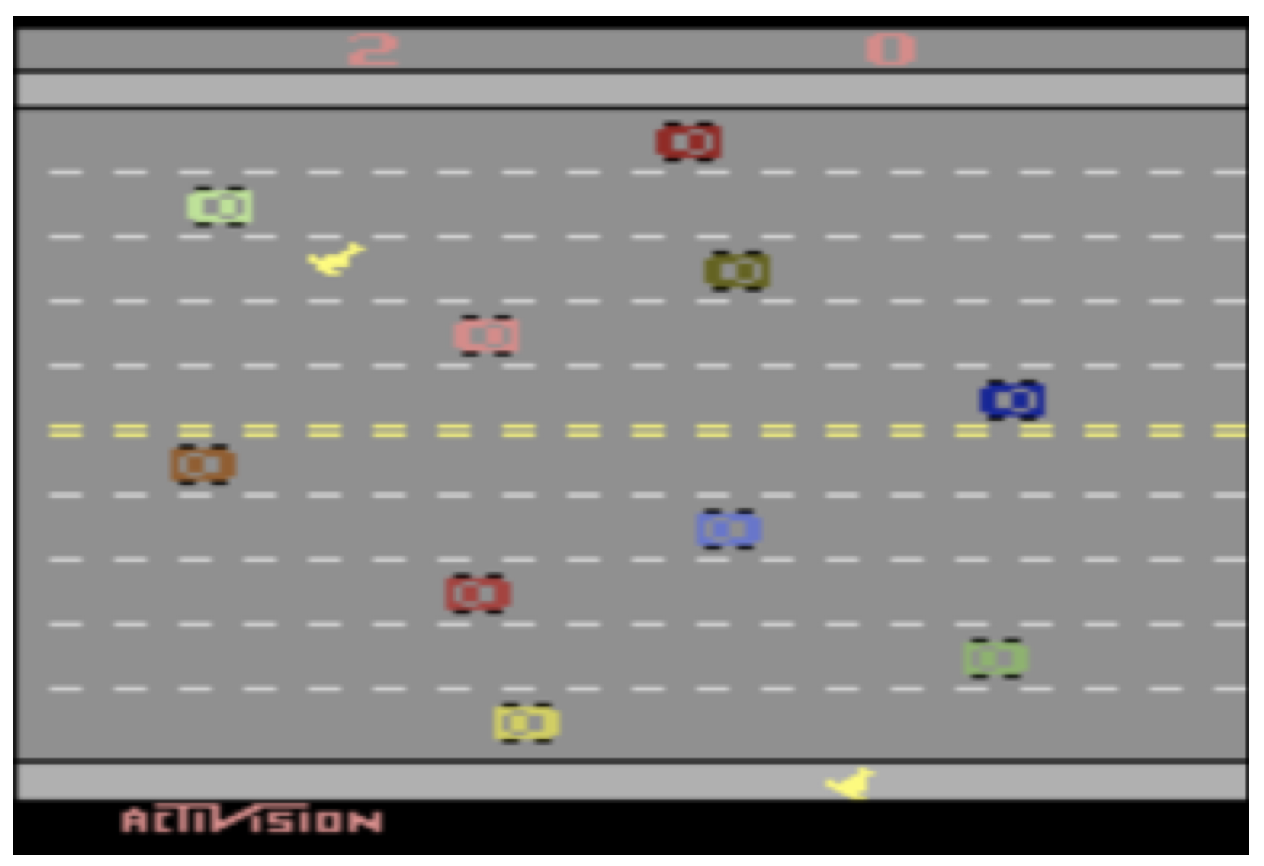# 人工智能自动规划 10：更高效的强化学习：回报设计和 Q-函数逼近

## 墨尔本大学 COMP90054 课程笔记

Posted by YEY on May 12, 2020

# Lecture 10 更高效的强化学习：回报设计和 $Q$-函数逼近

1. 动机
2. $Q$-函数逼近
3. 回报设计和初始化 $Q$-函数
4. 总结

## 1. 动机

### 1.1 学习成果

1. 给定一些已知特征，手动应用线性 $Q$-函数逼近来求解小规模 MDP 问题。
2. 选择合适的特征，设计并实现 $Q$-函数逼近，以实现无模型强化学习技术，从而自动求解中等规模的 MDP 问题。
3. 比较各种函数逼近方法的优缺点。
4. 比较和对比线性函数逼近与基于深度神经网络的函数逼近。
5. 说明如何使用回报设计来帮助无模型强化学习方法达到收敛。
6. 将回报设计手动应用于给定的潜在函数，以解决小规模 MDP 问题。
7. 设计并实现潜在函数，以自动解决中等规模的 MDP 问题。
8. 对比和比较回报设计与 $Q$-函数初始化。

### 1.2 强化学习：一些弱项

• 不同于蒙特卡洛方法中在获得回报后将该回报进行反向传播，TD 方法使用 bootstrapping（它们使用 $Q(s,a)$ 来估计未来折扣回报），这意味着对于回报稀疏问题，它可能需要很长时间才能将回报传播到整个 $Q$-函数。
• 两种方法都估计了一个 $Q$-函数 $Q(s,a)$，而对此最简单的建模方法是通过 $Q$-表。但是，这需要我们维护一个大小为 $|A|\times |S|$ 的表，而对于任何非平凡的问题来说，这样一张表都显得过于庞大了。
• 使用 $Q$-表 要求我们对于每个可到达的状态进行多次访问，并且对于每个行动应用多次以获得一个较好的 $Q(s,a)$ 的估计。因此，如果我们从未访问过某个状态 $s$，我们将无法估计 $Q(s,a)$，即便我们已经访问过一些与状态 $s$ 非常相似的状态。
• 回报可能非常稀疏，这意味着只有很少的状态/动作会得到非零回报。这显然是有问题的，因为初始时，强化学习算法的行为完全是随机的，并且很难发现那些较好的回报。还记得上一讲的 Freeway 游戏的演示吗？UCT 在那种情况下失效了。

### 1.3 强化学习：一些改进

• $n$-步时序差分学习：蒙特卡洛技术执行整个行动轨迹，然后反向传播回报；而基本的 TD 方法仅查看下一步的回报，来估计未来回报。相比之下，$n$-步方法在更新回报之前，将查看向前 $n$-步的回报，然后估算剩余部分。
• 逼近方法：相比计算一个精确的 $Q$-函数，我们可以使用简单方法来逼近它，这些方法既不需要大型 $Q$-表（因此方法的可扩展性更好），同时也可以用来提供合理的 $Q(s,a)$，即使我们之前从未在状态 $s$ 下应用过行动 $a$
• 回报设计（Reward shaping）和 $Q$-值初始化：如果回报是稀疏的，我们可以通过修改/增强回报函数，来奖励那些我们认为能够使我们更接近解（目标状态）的行为，或者我们可以猜测最优 $Q$-函数 并将其设为初始 $Q(s,a)$。

## 2. $Q$-函数逼近

### 2.1 问题：大的状态空间

1. 为每个 $s$ 和 $a$ 存储 $Q(s,a)$ 值（例如：在 $Q$-表中）是不可行的。
2. $Q$-值 的传播需要很长时间。

$480^2 + 2^{480} = 3.12\times 10^{144}$

### 2.2 特征选择练习: Freeway 游戏

Atari 2600 Freeway（高速公路）游戏中，为了帮助我们的算法学习如何使鸡成功穿越高速公路，我们应该选择哪些可能的较好特征？### 2.3 线性函数逼近

1. 对于状态，我们考虑能够确定其表示形式的特征是什么。
2. 在学习过程中，我们的更新是基于 特征的权重 而非状态本身。
3. 通过对特征进行加权求和来估计 $Q(s,a)$。

### 2.4 近似 $Q$-函数表示

• 特征向量 $f(s,a)$：它是一个由 $n\cdot |A|$ 个不同函数构成的向量，其中 $n$ 是状态特征数，$|A|$ 是行动数。每个函数都提取一个 “状态-行动” 对 $(s,a)$ 的特征值。我们说 $f_i(s,a)$ 从 “状态-行动” 对 $(s,a)$ 中提取了第 $i$ 个特征：

$f(s,a)=\begin{pmatrix} f_1(s,a) \\ f_2(s,a) \\ \dots \\ f_n(s,a) \end{pmatrix}$

Atari 2600 Freeway（高速公路）的例子中，我们有一个向量，该向量具有 $14$ 个状态特征乘以 $4$ 个行动。函数 $f_i(s,\textit{Up})$ 返回的是：如果选择向上移动，第 $i+1$ 行（对于 $12$ 行中的每一行，即 $i=1,\dots,12$）中距离最近的汽车；而函数 $f_{13}(s,\textit{Up})$ 返回的是第 $1$ 只鸡离目标的距离；函数 $f_{14}(s,\textit{Up})$ 返回的是第 $2$ 只鸡离目标的距离。例如：$f_{13}(s,a)=\frac{row(s,chicken)}{max_row}$。

• 一个大小为 $n\times |A|$ 的 权重向量 $w$：每个 “特征-行动” 对都具有一个权重。$w_i^a$ 定义了特征 $i$ 对于行动 $a$ 的权重。

### 2.5 定义 “状态-行动” 特征本作品采用知识共享署名-非商业性使用-相同方式共享 4.0 国际许可协议进行许可。 欢迎转载，并请注明来自：YEY 的博客 同时保持文章内容的完整和以上声明信息！